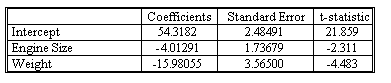### Why should I choose AnalystNotes?

AnalystNotes specializes in helping candidates pass. Period.

##### Subject 2. Testing the Significance of a Regression Coefficient
Let βk* denote some hypothesized value of the regression coefficient βk. If the basic assumptions hold, then the appropriate test statistic is

t = (bi - βk*)/sbi

Case 1: Suppose we wish to test the null hypothesis H0: βk = βk* or H0: βk ≤ βk* against the one-sided alternative hypothesis H1: βk > βk* using a level of significance α. Use the decision rule

Reject H0 in favor of H1 if t > tα, v

where the degrees of freedom of the Student t distribution is v = (T - K - 1).

Case 2: To test the null hypothesis H0: βk = βk* or H0: βk ≥ βk* against the one-sided alternative hypothesis H1: βk < βk*, use the decision rule

Reject H0 in favor of H1 if t < t α, v

Case 3: To test the null hypothesis H0: βk = βk* against the two-sided alternative hypothesis H1: βk ≠ βk*, use the decision rule

Reject H0 in favor of H1 if t < - tα/2, v or t > tα/2, v

Continue with the example in los a. It is reasonable to conjecture either that engine size has no effect on gasoline mileage or that the larger the engine, the lower the mileage. Thus, it is natural to test the null hypothesis H0: β1 = 0 against the one-sided alternative hypothesis H1: β1 < 0. Test this hypothesis using a 5% level of significance.

The data is shown below:The t-statistic column reports the results of a t-test on the hypothesis that the population value of the regression intercept or slope coefficient equals 0.
t-statistic = (estimated regression coefficient - hypothesized population value of the regression coefficient) / standard error of the regression coefficient
= estimated regression coefficient / standard error of the regression coefficient
= column 1 / column 2.

We have α = 0.05, T = 10, and K = 2. The appropriate degrees of freedom is v = 10 - 2 - 1 = 7. The critical value of t having 7 degrees of freedom is -t0.05, 7 = -1.895.
The hypothesized value is β1* = 0. To test H0, we calculate the t statistic t = (b1 - 0)/sb1 = -4.0129 / 1.737 = -2.31.

Because this observed t statistic is less than the critical value, it falls in the rejection region. Thus, if we use α = 0.05, we reject H0 in favor of H1. However, if we had used the slightly different level of significance α = 0.025, then the critical value of the test would have been -t0.025 = -2.365. In this case, the observed t statistic would have fallen in the acceptance region and we would not have rejected H0.

In the model, the variable X2 measures the weight of the car. We would expect heavy cars to get lower gasoline mileage than light cars, so it is natural to test H0: β2 = 0 against H1: β2 < 0. The appropriate t statistic is -4.48. This t statistic falls in the critical region if we use a 5% level of significance, so we reject the null hypothesis.

We showed that if we performed a one-tailed test using a 2.5% level of significance, we would fail to reject the null hypothesis that β1 = 0, but if we used a 5% level of significance we would reject the null hypothesis. Now the question is: Should we keep the variable X1 in the model or remove it and express gasoline mileage solely as a function of car weight? This is a difficult question to answer, and the decision depends partly on the opinions of the investigator. In los o when we discuss what is called the multicollinearity problem, we shall get more insight into why it is difficult to determine whether β1 = 0.

Learning Outcome Statements

c. formulate a null and an alternative hypothesis about the population value of a regression coefficient, calculate the value of the test statistic, and determine whether to reject the null hypothesis at a given level of significance;

d. interpret the results of hypothesis tests of regression coefficients;

CFA® 2023 Level I Curriculum, Volume 1, Module 2

User Comment
IIkDII what is r in this equation ?
rt2007 correlation coeeficient
Tony1234 Last paragraph - we should never accept the null hypothesis - always failed to reject it.
Oksanata what a strange definition of H alternative for b2 parameter...